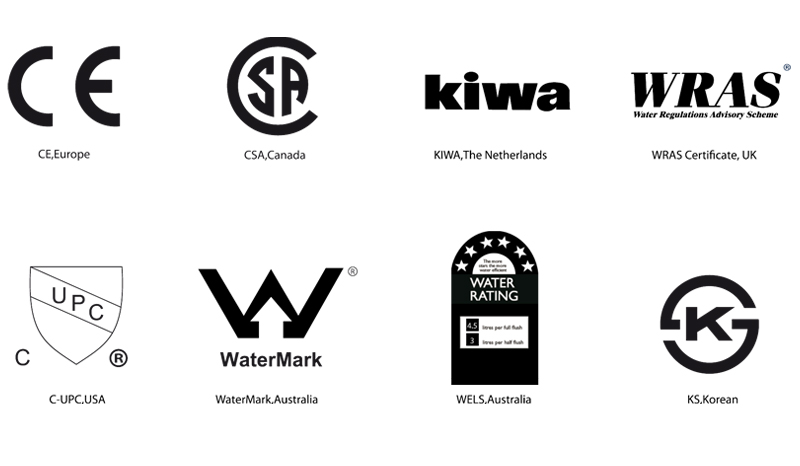# HK6812

RÉF: HK6812 Catégorie :
£1.00

## Description

size 600mm × 600mm × 9.8mm 4 pcs 32.5 kg 40 ctns 800 ctns 26000 kg
ITEM GB/T4100-2006 Appendix G
GB REQUIRED STANDARDS
HUIDA STANDARDS
The diviation of the average size
for each tile (2 sides)
from the work size
±0.5
n=10, Ac=0,,Re=2
-0.08~+0.06
d=0
The diviation in percent of the average
size for each tile(2 sides)
from the average size
of the 10 test specimens(20 sides)
±0.4
n=10, Ac=0,,Re=2
-0.01~+0.02
d=0
Thickness ±5
n=10, Ac=0,,Re=2
-1.0~+2.6
d=0
Staightness ±0.3
n=10, Ac=0,,Re=2
-0.02~+0.01
d=0
Wedging ±0.5
n=10, Ac=0,,Re=2
-0.07~+0.04
d=0
Central Bending Strenght(%) ±0.4
n=10, Ac=0,,Re=2
-0.02~+0.02
d=0
Edge Bending Strenght(%) ±0.4
n=10, Ac=0,,Re=2
-0.05~+0.03
d=0
Surface Quality a minimum of 95% of the tiles shall
be free from visible defects that
would impair the appearance of a
major area of tiles
n=5, Ac=0,,Re=2
no visible defects
d=0
Water Absorption average：E≤1
individual：E≤1
n=5, Ac=0,,Re=2
average：1
individual maximum：1
d=0
Disruptive Strenght thickness≥7.5mm,≥1300N
thickness≤7.5mm,≥700N
n=5, Ac=0,,Re=2
average value：3007
d=0
Broken Value(Mpa) average value：≥35MPa
individual：≥32MPa
n=5, Ac=0,,Re=2
average value：43
minimum value：42
d=0
Abrasion Resistance report abrasion class and
cycles passed
3 class（1500 cycles）
Thermal Expantion
Coeeficent
no crack or crazing after test
n=5, Ac=0,,Re=2
no crack or crazing
d=0
Frost Resistance no crack or crazing after test
n=10, Ac=0,,Re=2
no crack or crazing after test
d=0
Cracking Glaze
Strenght
no crazing on glazed surface after test
n=5, Ac=0,,Re=2
no crazing
d=0
Dirt
Resistance
minimum class 3
n=5, Ac=0,,Re=2
5
d=0
Resistance to household
chemicalsand swimming
pool salts
minimum GB
n=5, Ac=0,,Re=2
GA
d=0
Resistance to low
concentrations of acids
and alkalis
GLA、GLB、GLC GLA
Resistance to high
concentrations of acids
and alkalis
GHA、GHB、GHC GHA